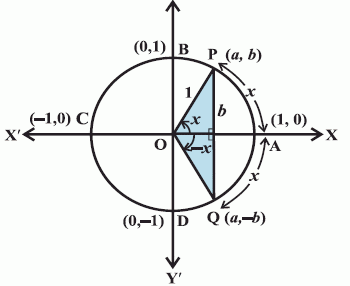Email us to get an instant 20% discount on highly effective K-12 Math & English kwizNET Programs!

#### Online Quiz (WorksheetABCD)

Questions Per Quiz = 2 4 6 8 10

### High School Mathematics - 210.8 Signs of Trigonometric Functions for Negative Angles

 θ sin θ cos θ tan θ cosec θ sec θ cot θ 0� 0 1 0 n.d. 1 n.d. 30� 1/2 �3/2 1/�3 2 2/�3 �3 45� 1/�2 1/�2 1 �2 �2 1 60� �3/2 1/2 �3 2/�3 2 1/�3 90� 1 0 n.d. 1 n.d. 0
Signs of trigonometric functions for negative angles
Let P(x,y) be a point on the unit circle with centre at origin such that angle AOP = θ. If angle AOQ = -θ, then the co-ordinates of the point Q will be (x,-y). Hence
cos(-θ) = x = cosθ
sin(-θ) = -y = -sinθI II III IV sinθ + + - - cosθ + - - + tanθ + - + - cosecθ + + - - secθ + - - + cotθ + - + -
In the first quadrant, as θ increases from 0 to π/2, sinθ increases from 0 to 1.
In the second quadrant, as θ increases from to π/2 to π,to π sinθ decreases from 1 to 0.
In the third quadrant, as θ increases from π to 3π/2, sinθ decreases from 0 to -1.
In the fourth quadrant, sinθ increases from -1 to 0, as θ increases from 3π/2 to 2π.

Directions: Solve the following.
 Q 1: State the quadrant in which cosec x = 4.1477 may lie.II, IIII, IVI, II Q 2: State the quadrants in which Sin x = .5732 may lie.I, IIII, IIII, IV Q 3: Given sec x = 8/5 and 3π/2 < x < 2π, evaluate cot x.15/85/88/5 Q 4: State the quadrants in which tan x is negative and cos x is positive.IIIIIIV Q 5: State the quadrant in which cot x = 0.8736 may lie.I, III, IVI, III Q 6: Given that sin x = 8/17 and x lies in quadrant I, find the value of tan x.8/178/1515/7 Q 7: Given sec x = 8/5 and 3π/2 < x < 2π, evaluate cosec x.- 8/(39)1/28/395/8 Q 8: If A lies in the second quadrant and tan x = -5/12, find the value of 2 cos x/(1-sin x)3-33/2 Question 9: This question is available to subscribers only! Question 10: This question is available to subscribers only!

#### Subscription to kwizNET Learning System offers the following benefits:

• Unrestricted access to grade appropriate lessons, quizzes, & printable worksheets
• Instant scoring of online quizzes
• Progress tracking and award certificates to keep your student motivated
• Unlimited practice with auto-generated 'WIZ MATH' quizzes